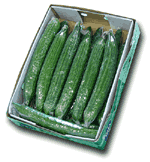Logic puzzles, riddles, math puzzles and brainteasers - pzzls.com

Vandaag is het 4 December 2023

Sort - Easiest on top
Page: << 7 8 9 10 11 >>

# Letter math - math puzzle

Difficulty:Rating: 3.2/5.0

```A - B = B
B * C = A
D : B = E
C * C = E
C + E = A
```

For which numbers stand A, B, C, D and E?

# Perfect numbers - math puzzle

Difficulty:Rating: 2.9/5.0

A perfect number is a number which is equal to the sum of all its divisors. For example 6 is divisable by 1,2,3 and 1 + 2 + 3 = 6. Can you find another perfect number?

# Cucumbers - math puzzle

Difficulty:Rating: 2.8/5.0

 In the morning a green-grocer puts 200 kg cucumbers outside in front of his shop. These cucumbers consists for 99% out of water. Because it is a warm day some water will evaporate. As a result, at the end of the day the cucumbers consist for 98% out of water. How many kg's of these cucumbers are left at the end of the day?